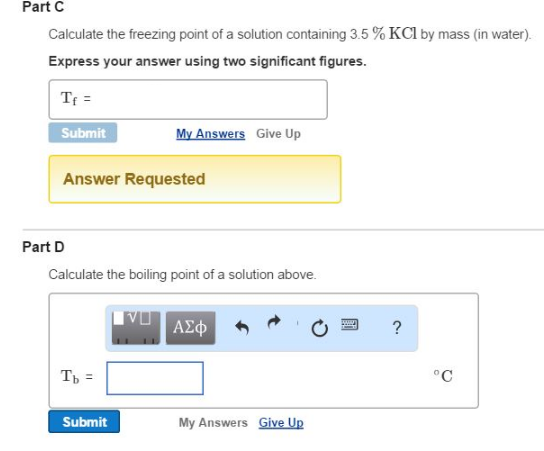# Problem: Part CCalculate the freezing point of a solution containing 3.5 % KCl by mass (in water). Express your answer using two significant figures. Part DCalculate the boiling point of a solution above.

🤓 Based on our data, we think this question is relevant for Professor Johnson's class at Southwestern Oklahoma State University.

###### FREE Expert Solution###### Problem Details

Part C

Calculate the freezing point of a solution containing 3.5 % KCl by mass (in water).

Part D

Calculate the boiling point of a solution above.